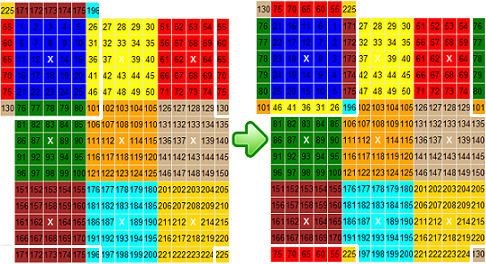HomePuzzle ContestSingle Shift PuzzleFlip Flat RubikDouble Shift PuzzleRotate 3x3 PuzzleRotate 5x5 PuzzleRotate 7x7 PuzzleNine Faces RubikSix Faces Rubik24 Faces Magic Cube4D Rubik CubeHow To Use Sudoku SolverSudoku RulesSudoku HistoryNaked SingleConflicting CandidatesNaked PairsNaked TriplesHidden SinglesHidden PairsPointing PairsBox/Line ReductionChainsQuotes By AuthorQuotes By SubjectQuote Of The DayDetective MysteryReversiLogic TestMath TestWord Of The DayPuzzlesUnsolved ProblemsHall of FameContact UsLoginSign UpSign OutMy AccountChange PasswordAbout UsContributorsLink To UsPrivacy PolicyTerms of ServiceSite Map

## Flat Rubik - Rotate 7x7 Puzzle

Rotate 7x7 Puzzle is an extension of 'Rotate 5x5' puzzle that consists of nine 5x5 color bocks. For the puzzle to be solved, colors should be separated and each block must be a solid color (see the left image in Figure 1).
There are 9 square marked with white “X”. By clicking on the white “X” square, you can rotate a 7x7 square block or 9x9 ring 90 degrees. There are two options: 'Rotate 7x7 Square Clockwise', 'Rotate 7x7 Square Counterclockwise', 'Rotate 9x9 Ring Clockwise', and 'Rotate 9x9 Ring Counterclockwise'.
For example, if you select the 'Rotate 7x7 Square Clockwise' option and click on white “X” square between squares #112 and #114 (an intersection of column #8 and row #8), 7x7 square block around white “X” square will rotate clockwise 90 degrees (see Figure 1). The 7x7 square block includes all squares between row #4 and row #12 and between column #4 and column #12 (three rows up and three rows down, and three columns left and three columns right from the white “X” square).Figure 1
If you select the 'Rotate 9x9 Ring Clockwise' option and click on white “X” square between squares #112 and #114 (an intersection of column #8 and row #8), perimeter of the 9x9 square block around white “X” square will rotate clockwise 90 degrees (see Figure 2).Figure 2
Columns are enumerated from #1 to #16. Next column after column #16 is a column #1 again. The previous column for column #1 is column #16. The same rule applies to the rows.Figure 3
For example, if you select the 'Rotate 7x7 Square Clockwise' option and click on white “X” square between square #12 and #14 (an intersection of column #3 and row #3), 7x7 square block around white “X” square will rotate clockwise 90 degrees (see Figure 3). The 7x7 square block includes all square three rows up and three rows down and, three columns left and three columns right from the white “X” square. It includes 7x7 square between the row #1 and row #4 and between column #1 and column #4, and also squares 225, 171, 172, 173, 174, and 196 from row #16, and squares 55, 60, 65, 75, and 130 from column #16.
Send us your Flat Rubik 9x5x5 Square Puzzle solution to become a member of our “Hall of Fame”.